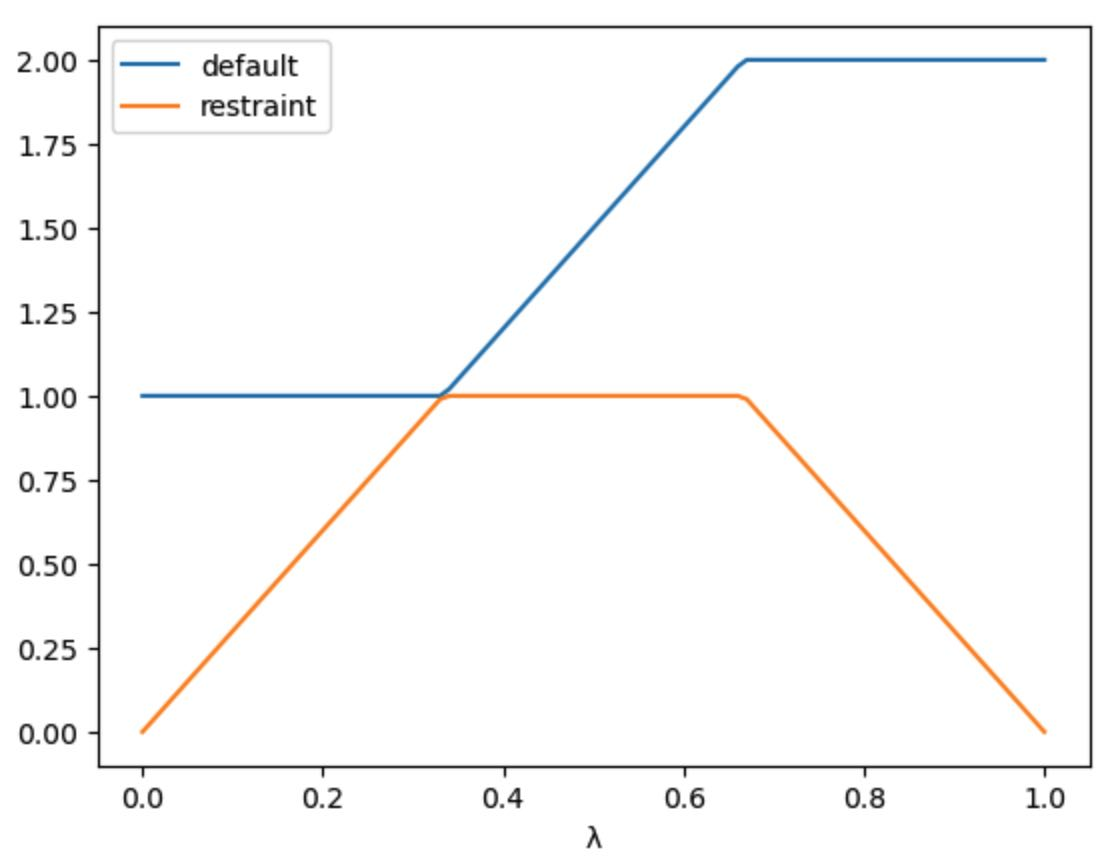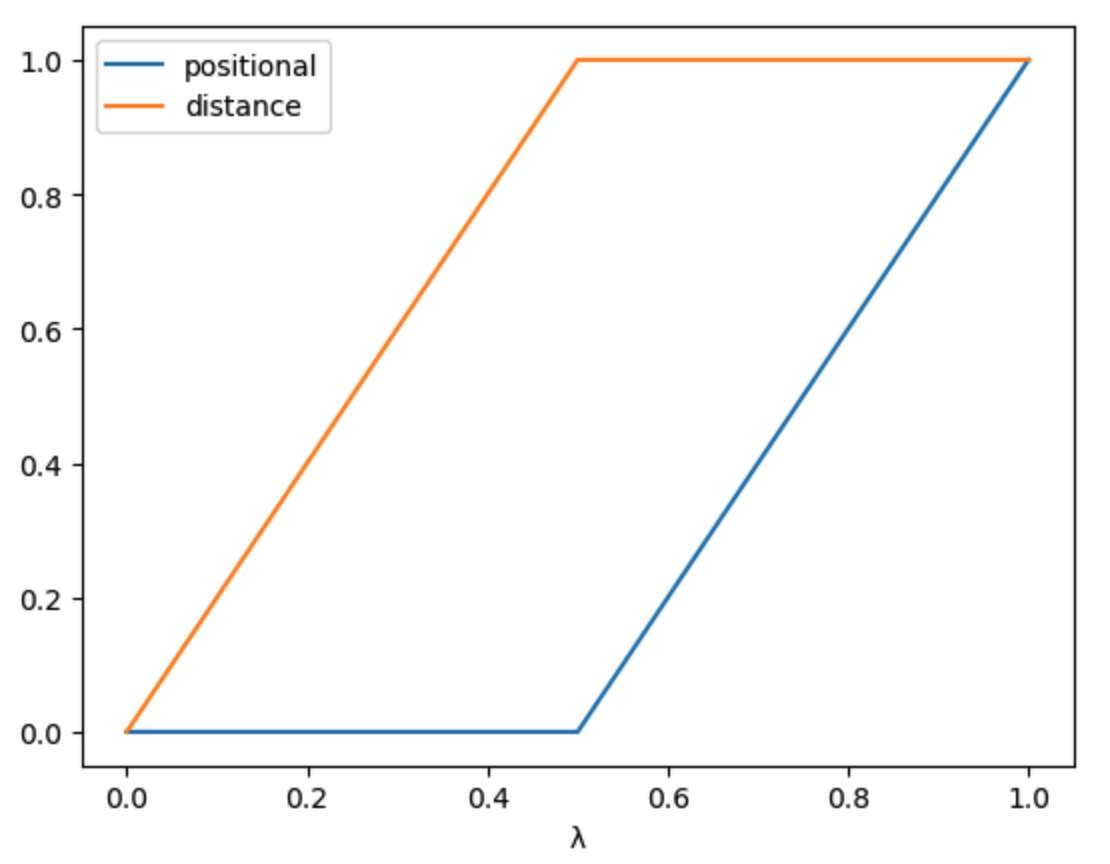# Alchemical Restraints#

You can perturb restraints as part of an alchemical free energy simulation. This is useful for computing free energy differences between two systems where you want to turn on or off restraints that are used to keep part of the molecules in place while you are performing the mutation.

You can perturb restraints by adding them to the `LambdaSchedule` used to control the perturbation.

By default, restraints are called `restraint`, and so are perturbed using the `restraint` lever.

```>>> import sire as sr
>>> l = sr.cas.LambdaSchedule()
>>> l.add_stage("morph", (1-l.lam()) * l.initial() + l.lam() * l.final())
>>> print(l)
LambdaSchedule(
restraints_on: initial
morph: initial * (-λ + 1) + λ * final
restraints_off: final
)
```

This has created a schedule with three stages, `restraints_on`, `morph` and `restraints_off`. The `morph` stage is the one that will be used to peturb the molecule from the initial state to the perturbed state.

The `restraints_on` and `restraints_off` stages will be used to switch on and off the restraints. To actually switch them on and off, we need to set the equation that will be used to scale the restraints.

```>>> l.set_equation("restraints_on", "restraint", l.lam() * l.initial())
>>> l.set_equation("restraints_off", "restraint", (1-l.lam()) * l.initial())
```

This will scale the restraints by λ in the `restraints_on` stage. This means that the restraints start at λ=0 scaled to 0, and are then fully switched on by λ=1.

The restraints are scaled off by (1-λ) in the `restraints_off` stage. This means that the restraints are fully switched on at λ=0, but then scaled down so that they are scaled to 0 at λ=1.

The value of `initial` and `final` for a restraint is the same, being 100% of the restraint. Thus `(1-l.lam()) * l.initial() + l.lam() * l.final()` will always evaluate to 100% of the restraint for all values of λ during the `morph` stage. You can make sure that the restraint is kept at 100% during this stage by setting this value explicitly;

```>>> l.set_equation("morph", "restraint", l.initial())
>>> print(l)
LambdaSchedule(
restraints_on: initial
restraint: λ * initial
morph: initial * (-λ + 1) + λ * final
restraint: initial
restraints_off: final
restraint: final * (-λ + 1)
)
```

You can view the effect of this schedule by plotting the perturbation assuming an initial value of `1.0` and a final value of `2.0` using

```>>> l.get_lever_values(initial=1.0, final=2.0).plot()
```Here we can see the three stages of the schedule. The `restraints_on` stage keeps the `default` value of the restraint at the initial value (`1.0`) while it scales the restraint from `0` to `1.0`. The `morph` stage keeps the restraint at `1.0`, while scaling the parameter from `1.0` to `2.0`. Finally, the `restraints_off` stage scales the restraint from `1.0` to `0` while keeping the parameter at `2.0`.

We can now use this schedule to perturb the restraints during an alchemical simulation e.g.

```>>> mols = sr.load(sr.expand(sr.tutorial_url, "merged_molecule.s3"))
>>> restraints = sr.restraints.positional(mols, "molidx 0")
>>> mols = mols.minimisation(restraints=restraints,
...                          lambda_value=0.0).run().commit()
>>> d = mols.dynamics(timestep="4fs", temperature="25oC",
...                   restraints=restraints, schedule=l,
...                   lambda_value=0.0)
>>> d.run("10ps")
>>> mols = d.commit()
```

## Using named restraints#

By default, all restraints in a system are called `restraint`, and so are perturbed using the `restraint` lever. However, you can also give restraints their own name, and then perturb them using their name. For example, here we create two restraints, named `positional` and `distance`.

```>>> pos_rest = sr.restraints.positional(mols, "molidx 0", name="positional")
>>> dst_rest = sr.restraints.distance(mols, atoms0=0, atoms1=1, name="distance")
>>> print(pos_rest, dst_rest)
PositionalRestraints( name=positional, size=8
0: PositionalRestraint( 0 => ( 25.7128, 24.9375, 25.2539 ), k=150 kcal mol-1 Å-2 : r0=0 Å )
1: PositionalRestraint( 1 => ( 24.2872, 25.0626, 24.7461 ), k=150 kcal mol-1 Å-2 : r0=0 Å )
2: PositionalRestraint( 2 => ( 25.9115, 23.8899, 25.5639 ), k=150 kcal mol-1 Å-2 : r0=0 Å )
3: PositionalRestraint( 3 => ( 26.425, 25.2206, 24.4509 ), k=150 kcal mol-1 Å-2 : r0=0 Å )
4: PositionalRestraint( 4 => ( 25.8616, 25.6094, 26.1259 ), k=150 kcal mol-1 Å-2 : r0=0 Å )
5: PositionalRestraint( 5 => ( 24.1384, 24.3907, 23.8741 ), k=150 kcal mol-1 Å-2 : r0=0 Å )
6: PositionalRestraint( 6 => ( 24.0888, 26.1101, 24.4351 ), k=150 kcal mol-1 Å-2 : r0=0 Å )
7: PositionalRestraint( 7 => ( 23.575, 24.7795, 25.5491 ), k=150 kcal mol-1 Å-2 : r0=0 Å )
) BondRestraints( name=distance, size=1
0: BondRestraint( 0 <=> 1, k=150 kcal mol-1 Å-2 : r0=1.5185 Å )
)
```

We can now create a schedule that perturbs these restraints separately using their names. We will first scale up the `distance` restraint in a `distance_restraints` stage…

```>>> l = sr.cas.LambdaSchedule()
>>> l.set_equation("distance_restraints", "distance", l.lam() * l.initial())
```

and will then scale up the `positional` restraint in a `positional_restraints` stage, while keeping the `distance` restraint fully on.

```>>> l.add_stage("positional_restraints", 1)
>>> l.set_equation("positional_restraints", "positional", l.lam() * l.initial())
>>> print(l)
LambdaSchedule(
distance_restraints: 0
distance: λ * initial
positional_restraints: 1
positional: λ * initial
)
>>> l.get_lever_values(initial=1.0, final=1.0,
...                    levers=["positional", "distance"]).plot()
```Here we can see that, in the first `distance_restraints` stage (from λ=0 to λ=0.5), the `distance` restraint is scaled from `0` to `1` while the `positional` restraint is kept at `0`. In the second `positional_restraints` stage (from λ=0.5 to λ=1), the `positional` restraint is scaled from `0` to `1` while the `distance` restraint is kept at `1`.

We can now use this schedule in a simulation, e.g.

```>>> mols = sr.load(sr.expand(sr.tutorial_url, "merged_molecule.s3"))
>>> mols = mols.minimisation(restraints=[dst_rest, pos_rest],
...                          lambda_value=0.0).run().commit()
>>> d = mols.dynamics(timestep="4fs", temperature="25oC",
...                   restraints=[dst_rest, pos_rest], schedule=l,
...                   lambda_value=0.0)
>>> d.run("10ps")
>>> mols = d.commit()
```